Next: Plotting with Maple Up: Preliminaries about Maple Previous: The int (and Int)

Subsections

# The subs command

You have learned in the previous sections that if you assign a particular value to a variable, Maple will remember it for the rest of the session until you unassign it. At times, you might like to plug in some values into an expression without altering the variable (or variables) involved. Maple has the subs command which reports the result of making such a substitution:
>
y:=x+3;>
subs(x=2,y);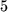Notice that Maple reports the value obtained when plugging in x=2 into y without giving that value to x or altering y in any way:

>
x;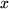>
y;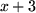You can make more than one substitution:

>
z:=x+sin(2*h):
subs(x=3*u+1,h=4*Pi*q,z);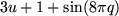In this example, the first assignment is ended with a colon : to suppress the display. You can do this whenever you want to make an assignment which you do not want to see displayed. Then, plugging x = 3u + 1 and h = 4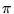q into the expression for z, we obtain 3u + 1 + sin(8q). Notice that the values of x and h that we plug in do not need to be enclosed in braces when issuing the command.

You should be aware of a few things when using the subs command:

• You need not fear circular substitutions:
>
z;>
subs(x=2*x,z);On the other hand, long chains of self-referential substitutions may produce results which are hard to predict:

>
subs(h=x,x=z,z);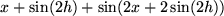Simultaneous substitutions are made enclosing the values you plug in in braces. This is in contrast with the left-to-right substitution above:

>
subs(h=x,x=z,z);• In certain situations, subs will not be able to find the expression you are substituting for if it is complicated. In those cases, try to reword your substitution request so that Maple understands it, or break the task into several manageable ones. In other cases, you might consider using algsubs, which allows you to substitute one algebraic expression for another.

• subs does what is called syntactic substitution''; it substitutes one set of symbols for another. If you are using it to evaluate an expression with certain values plugged in'', using eval might be more appropriate. Here is an example that should illustrate the distinction:

>
integral := int(f(t,a), t=a..x);>
eval(integral,t=0,a=1);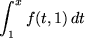>
subs(t=0,a=1,integral);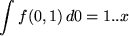The seemingly nonsensical answer using subs above is because Maple interpreted the expression as meaning int(f(0,1),0=1..x) -- what you get by replacing t by 0 and a by 1 without trying to interpret the expression.

## Why is subs useful?

Often it is better to use subs rather than to make assignments to the variables in an expression. For example, if
>
y:=3*x^2+5*x+2;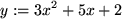and you want to compute the difference quotient (y(x + h) - y(x))/h, then you should execute

>
dq:=(subs(x=x+h,y)-y)/h;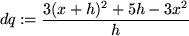because if we set

>
x:=x+h;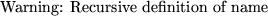any call to y will result in

>
y;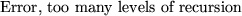In earlier versions of Maple, this action would have crashed your session.

On the other hand,

>
x:=4;>
y;is alright, but now y is a number and we cannot plug any other values of x into it. Using subs is a better way to do the plugging in, because we need not unassign y.Next: Plotting with Maple Up: Preliminaries about Maple Previous: The int (and Int)

Translated from LaTeX by Scott Sutherland
2002-08-29